# Frank Solutions for Class 10 Maths Chapter 12 Distance and Section Formulae

Frank Solutions for Class 10 Maths Chapter 12 Distance and Section Formulae, provided here in PDF format, are available for download. The main aim of creating these solutions is to help students understand and crack Maths problems. Subject experts at BYJU’S formulate these exercises to assist you with your exam preparation to attain good marks in Maths. Students who wish to score excellently in the subject should practise Frank Solutions for Class 10 Maths Chapter 12 diligently. The book is one of the top materials when it comes to providing a question bank to practise.

Chapter 12 – Distance and Section Formulae explains the distance formula, which is used to find the distance between two defined points on a graph. The section formula tells us the coordinates of the point, which divides a given line segment into two parts. Students will learn different related topics in this chapter.

## Download the PDF of Frank Solutions for Class 10 Maths Chapter 12 Distance and Section Formulae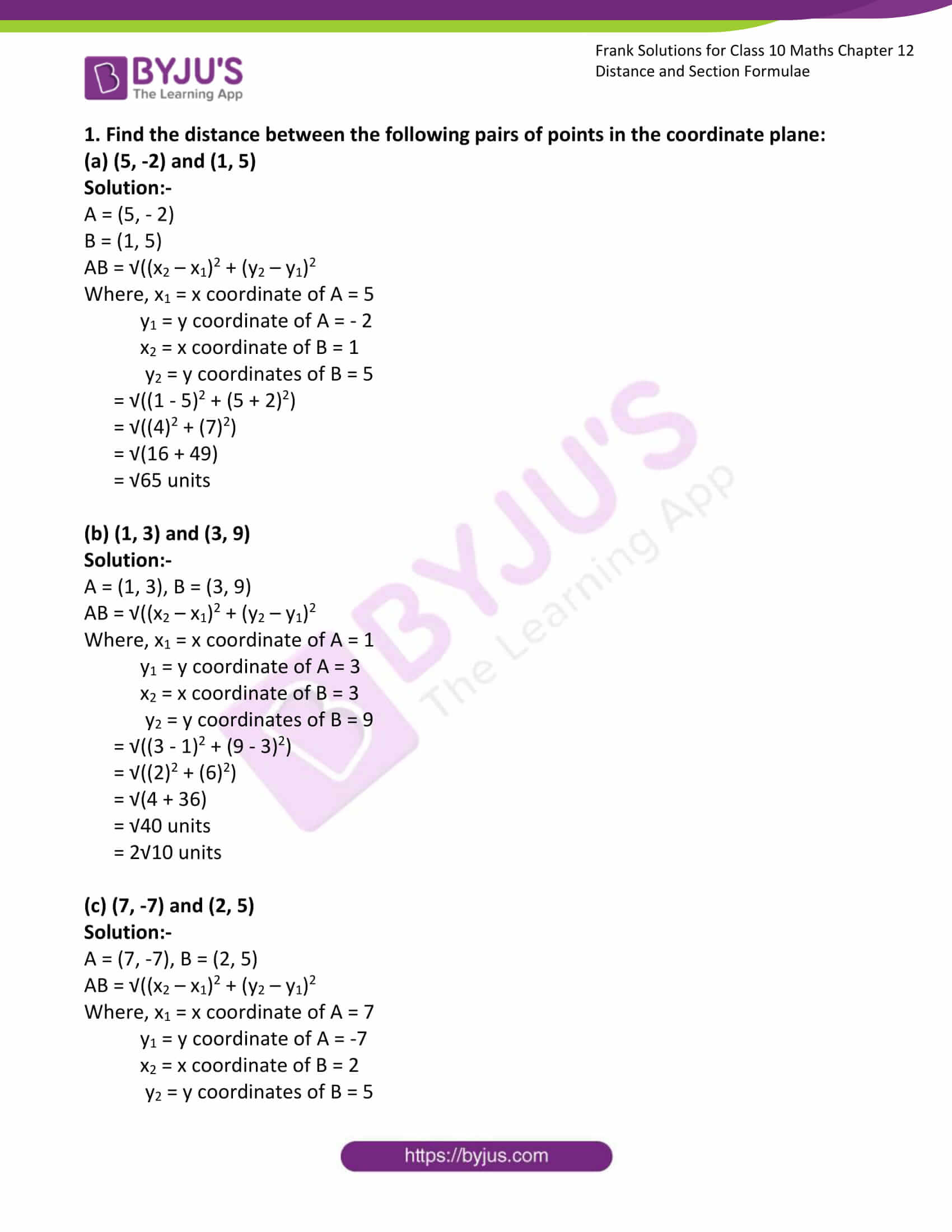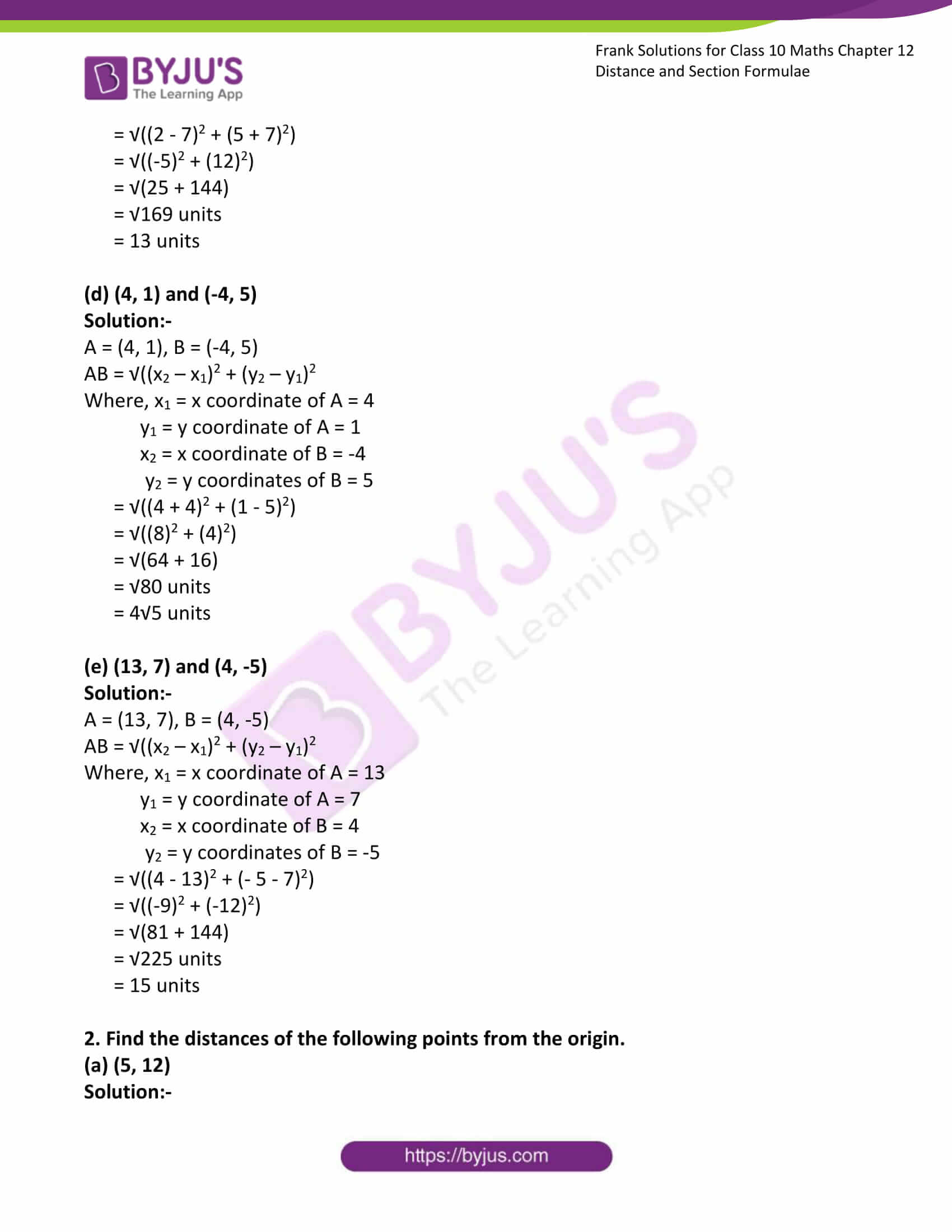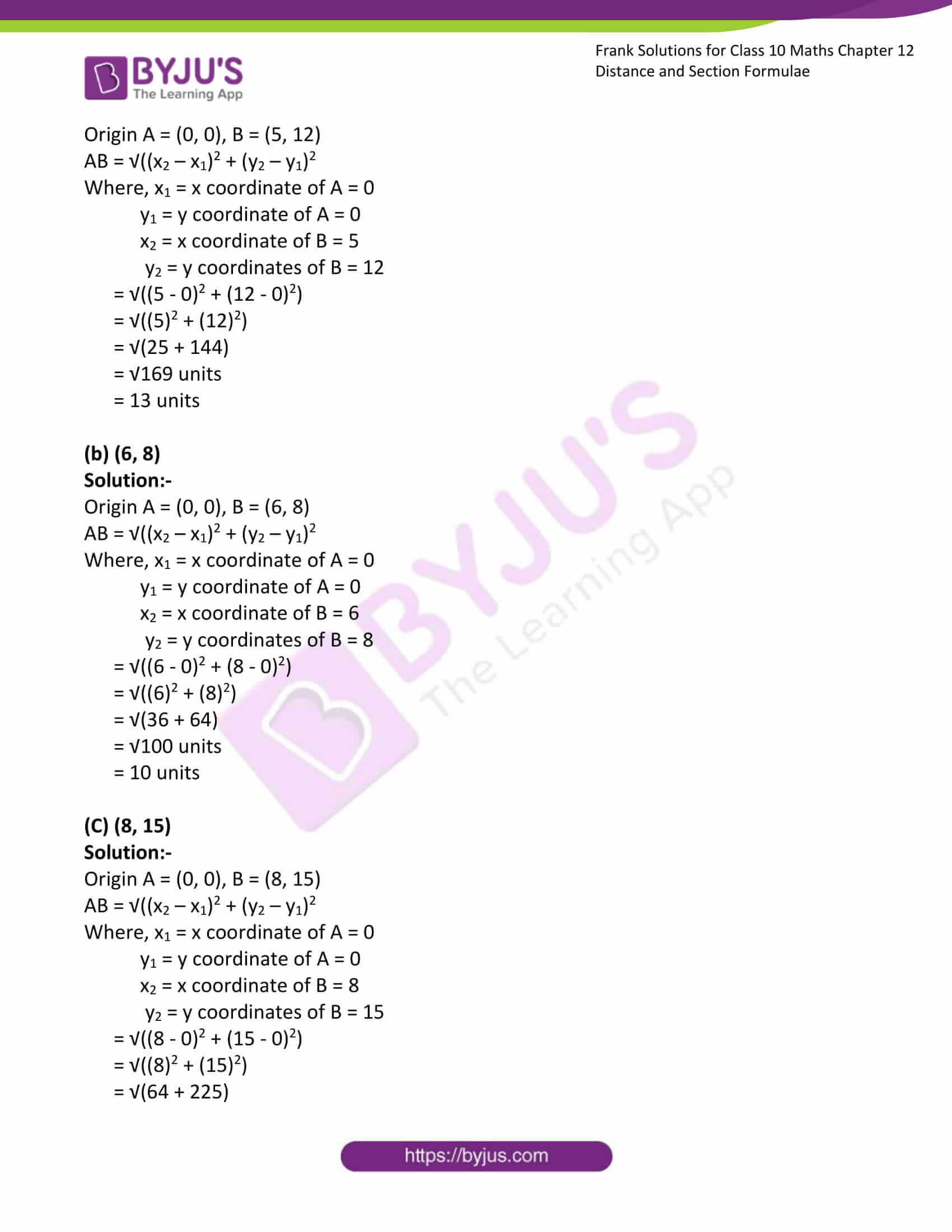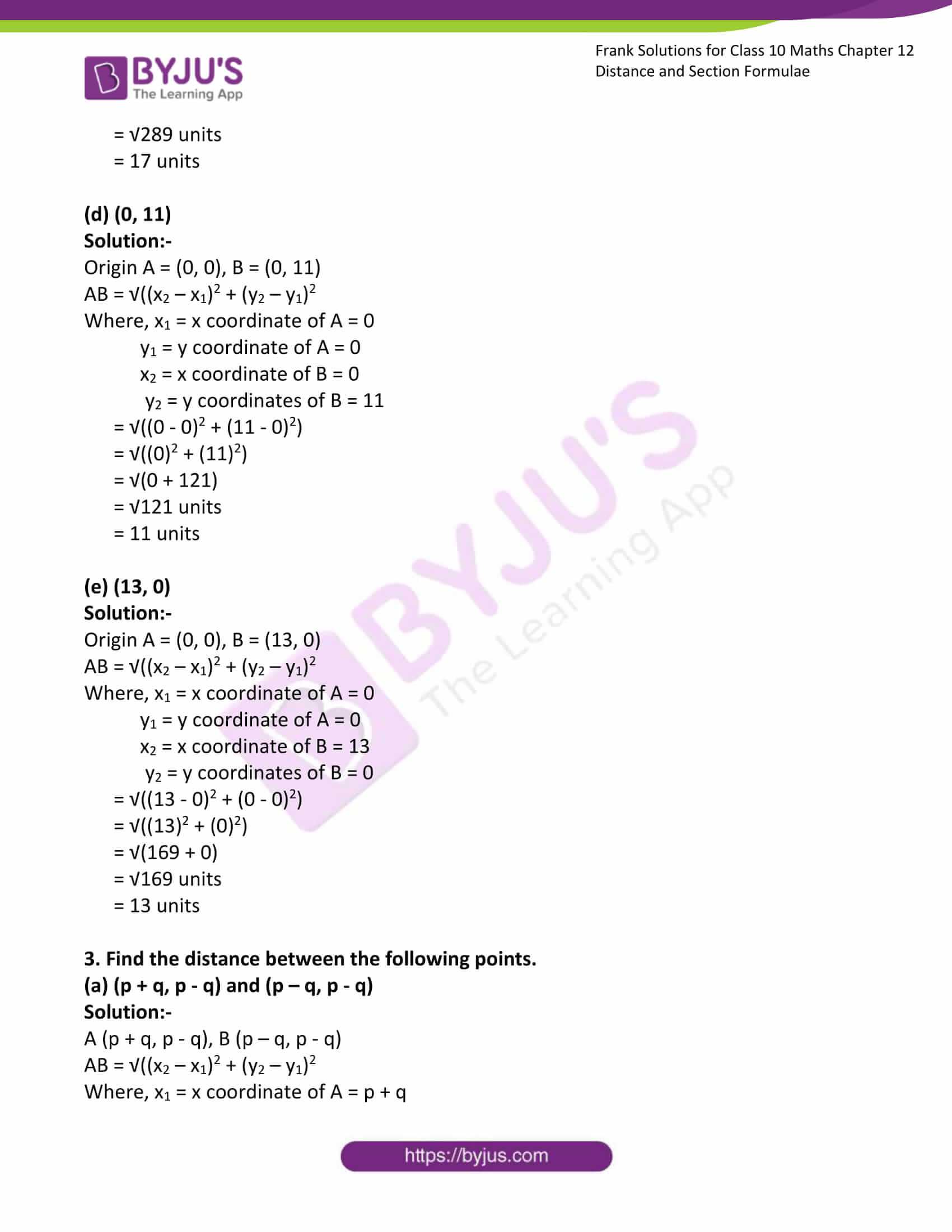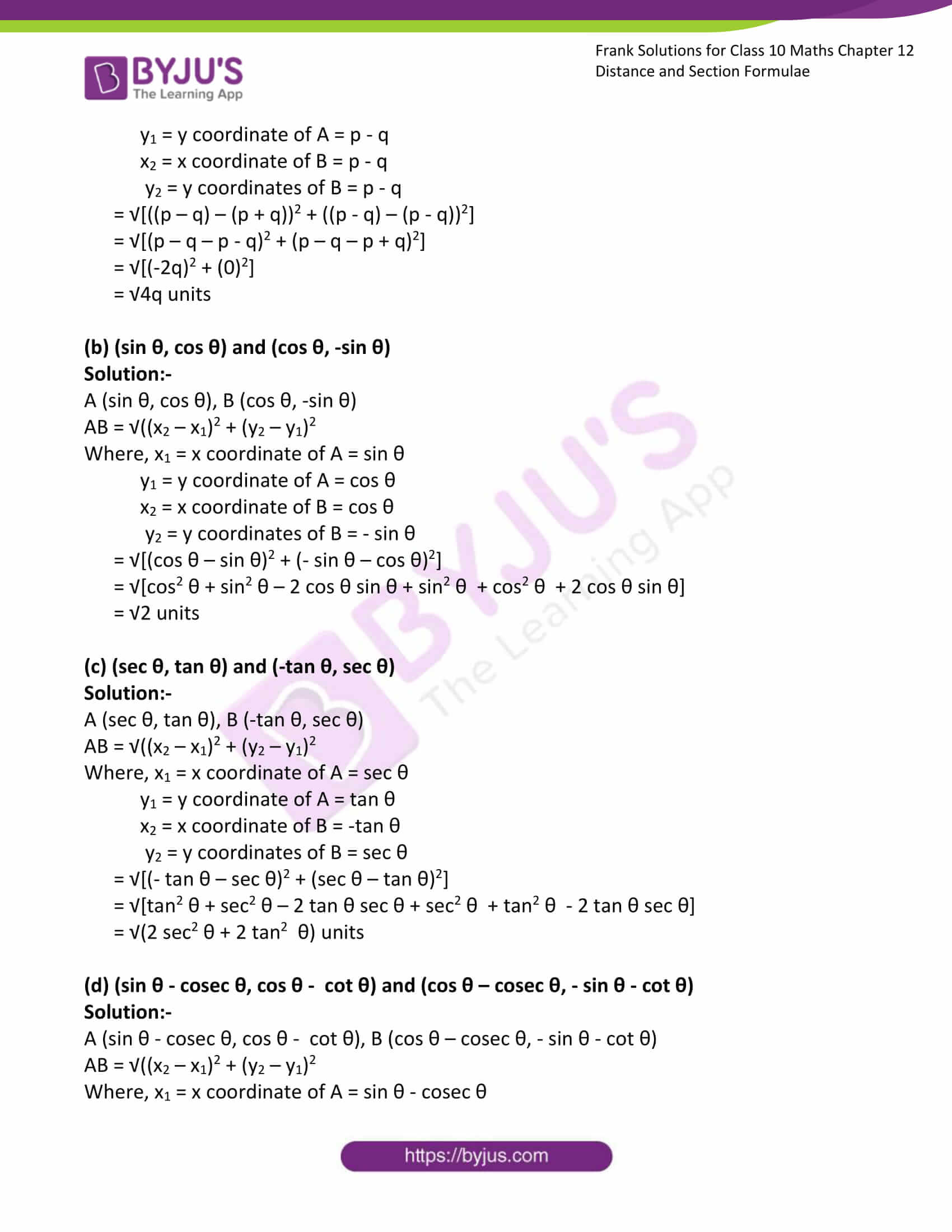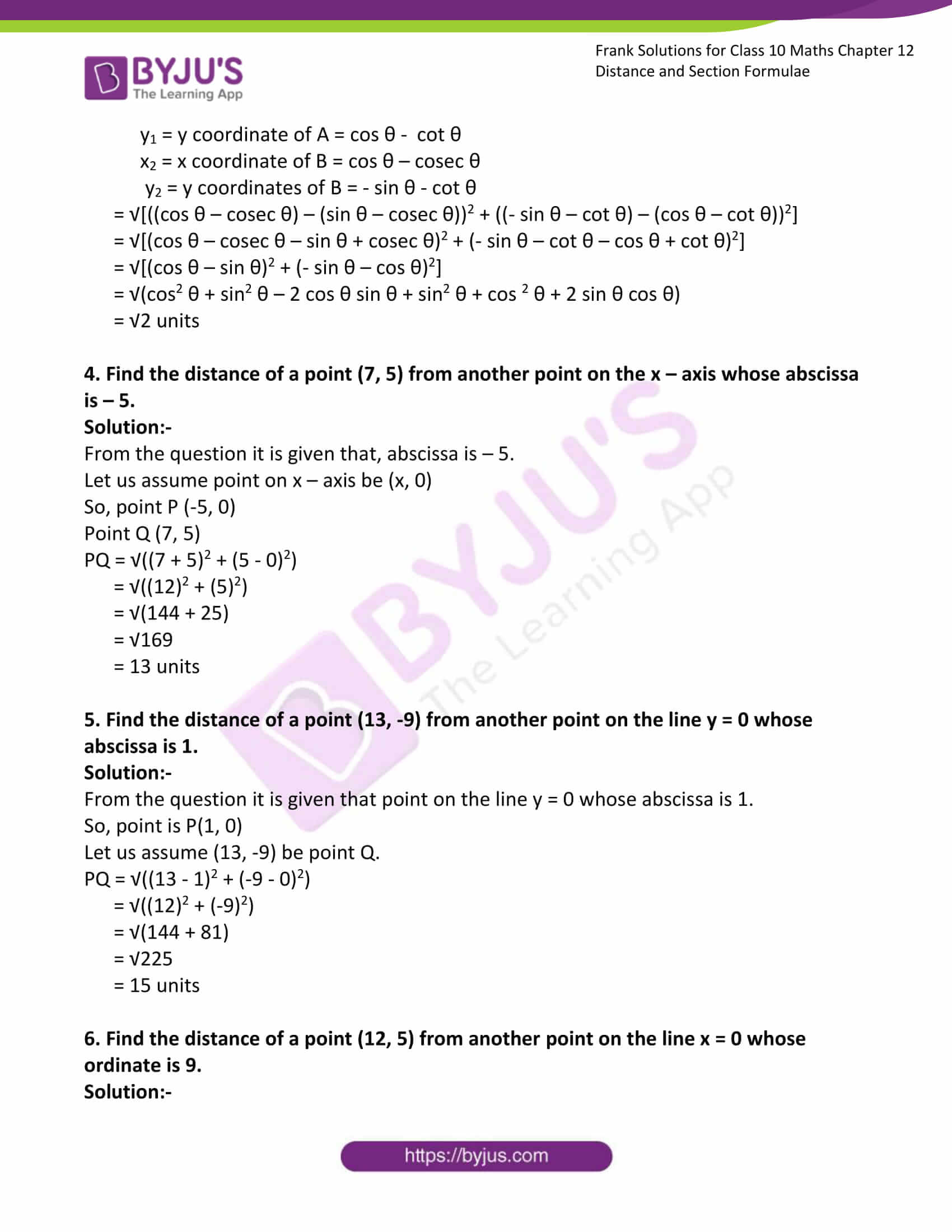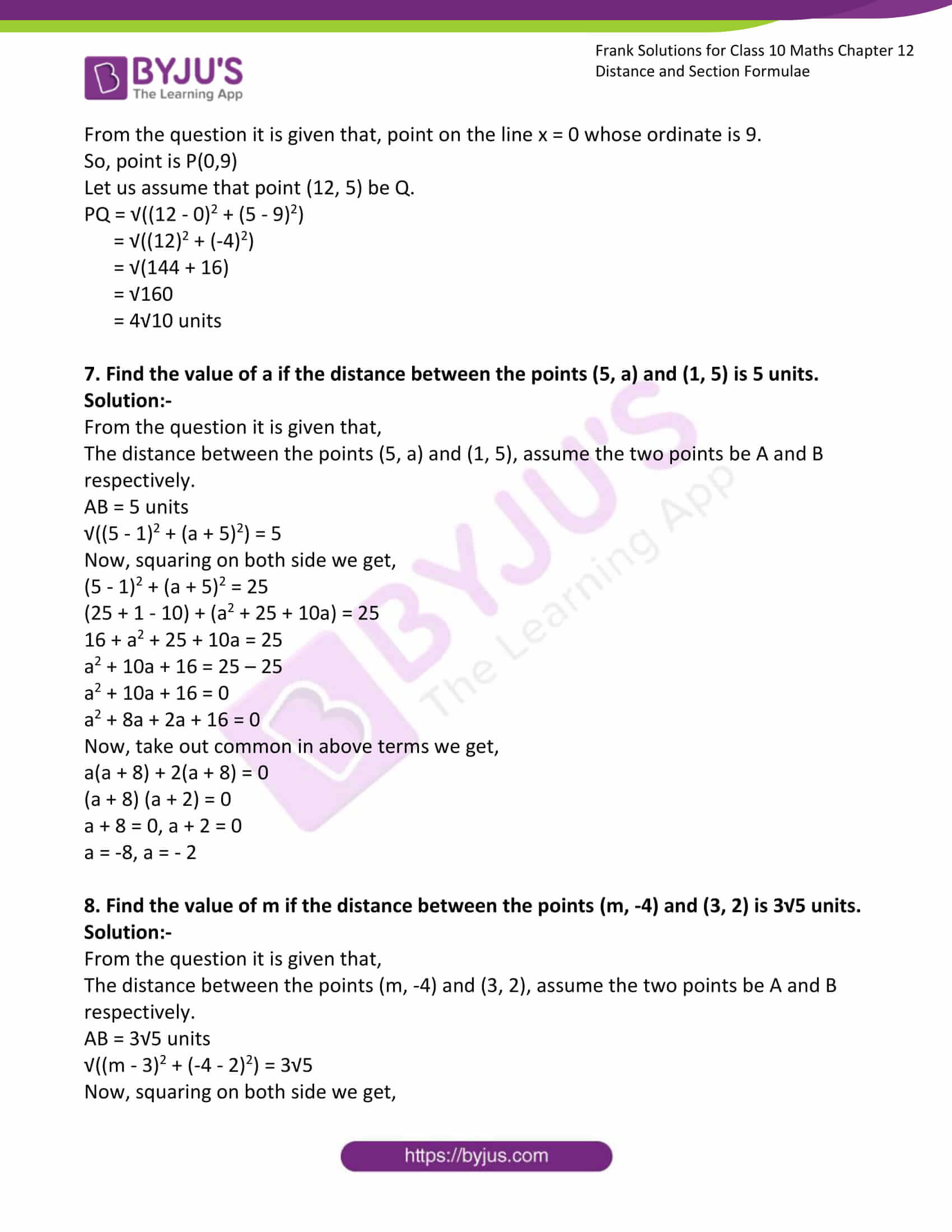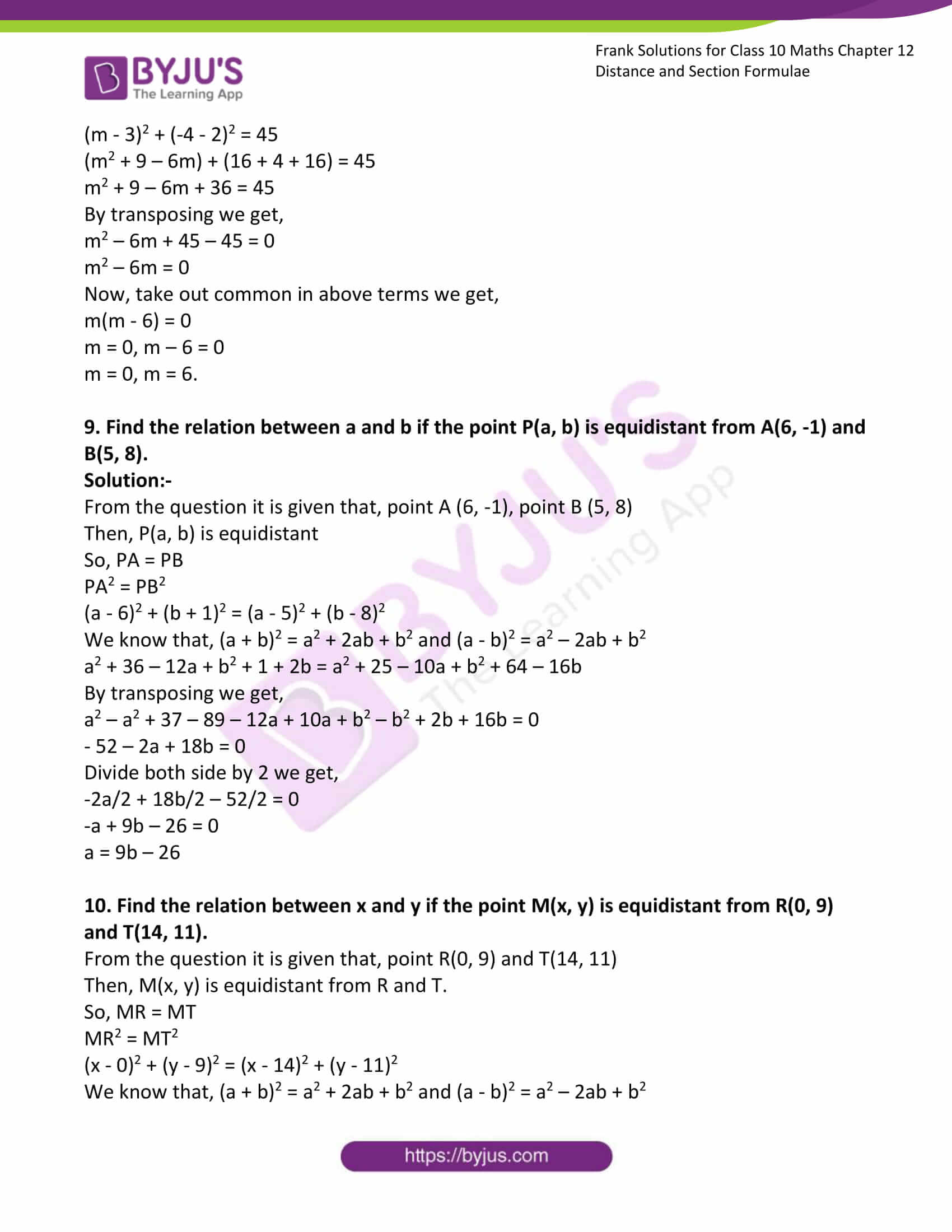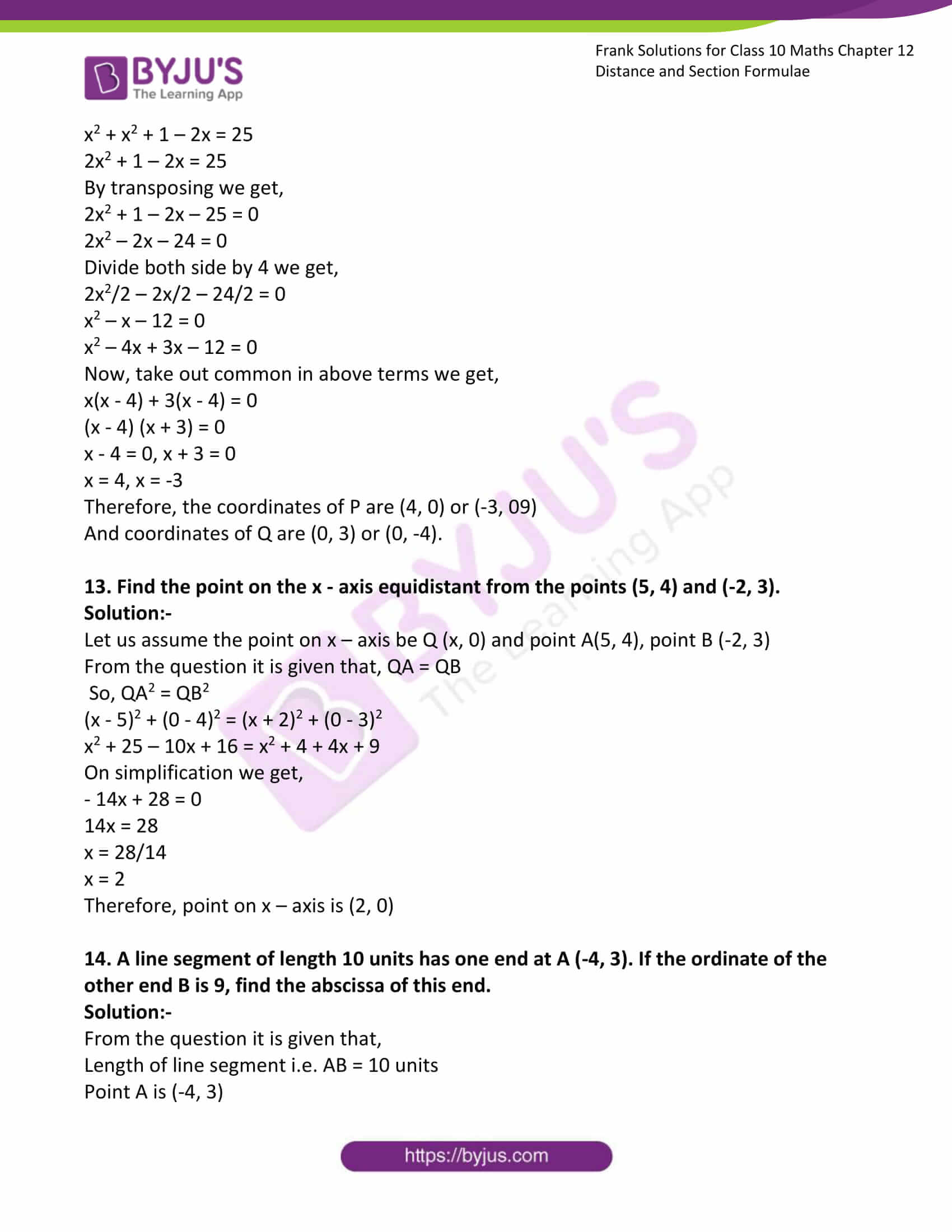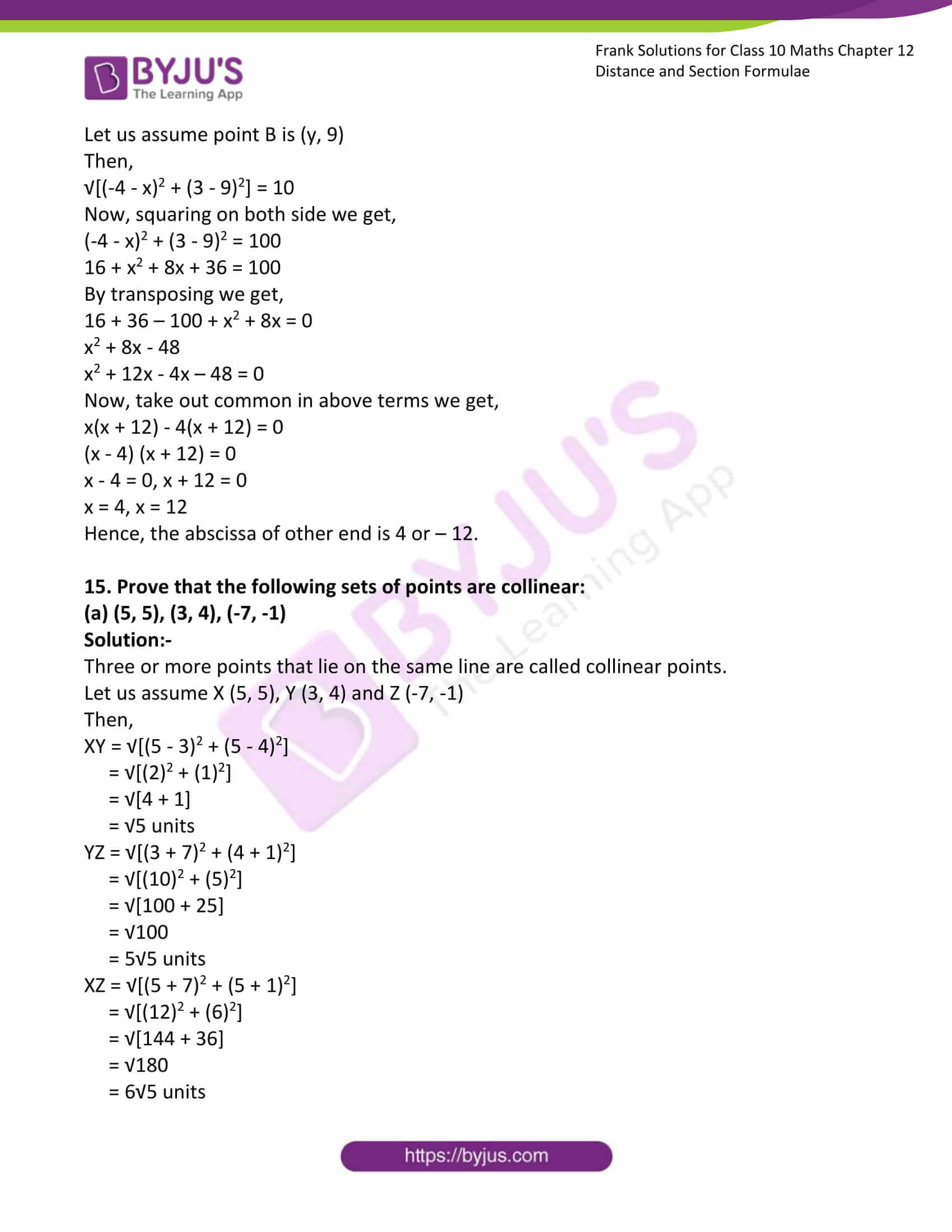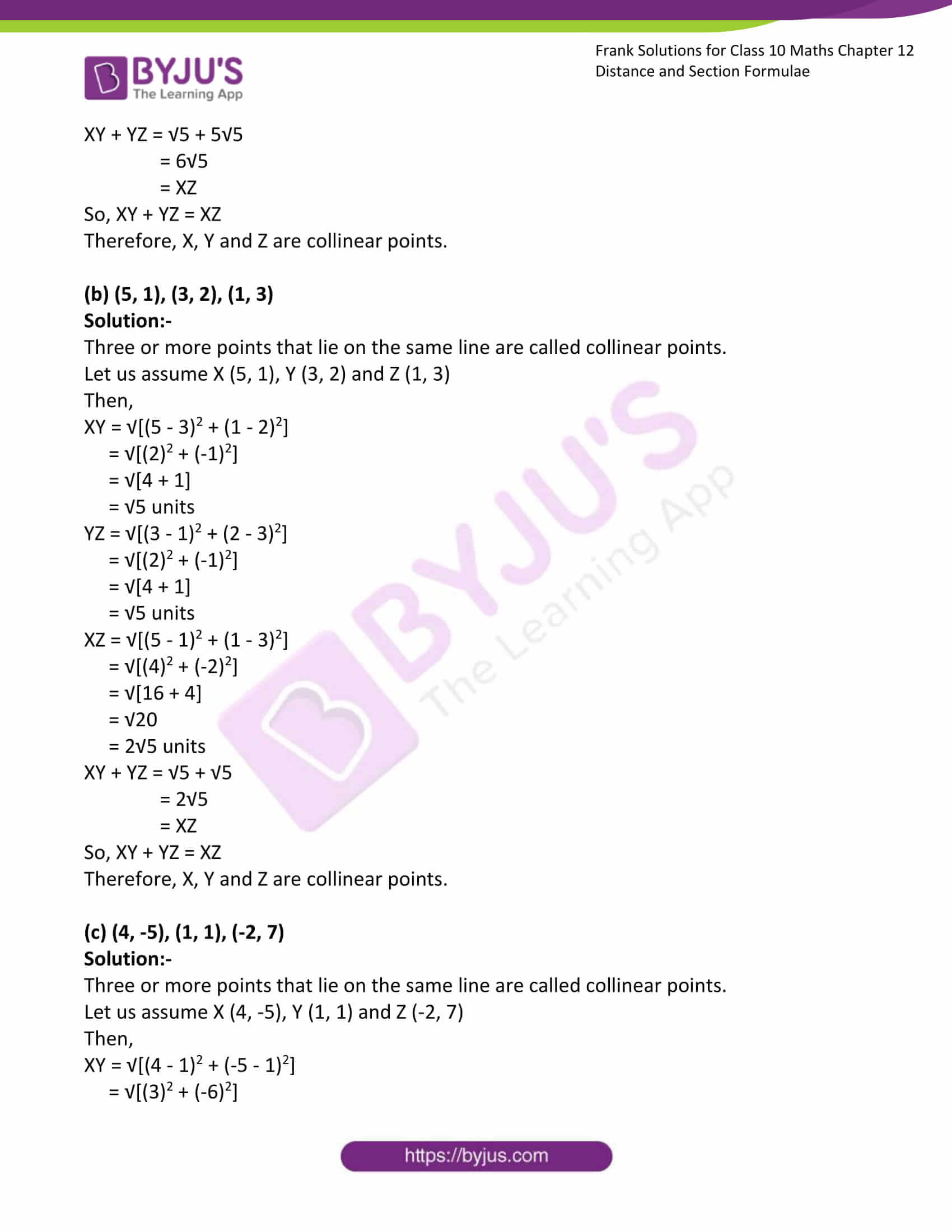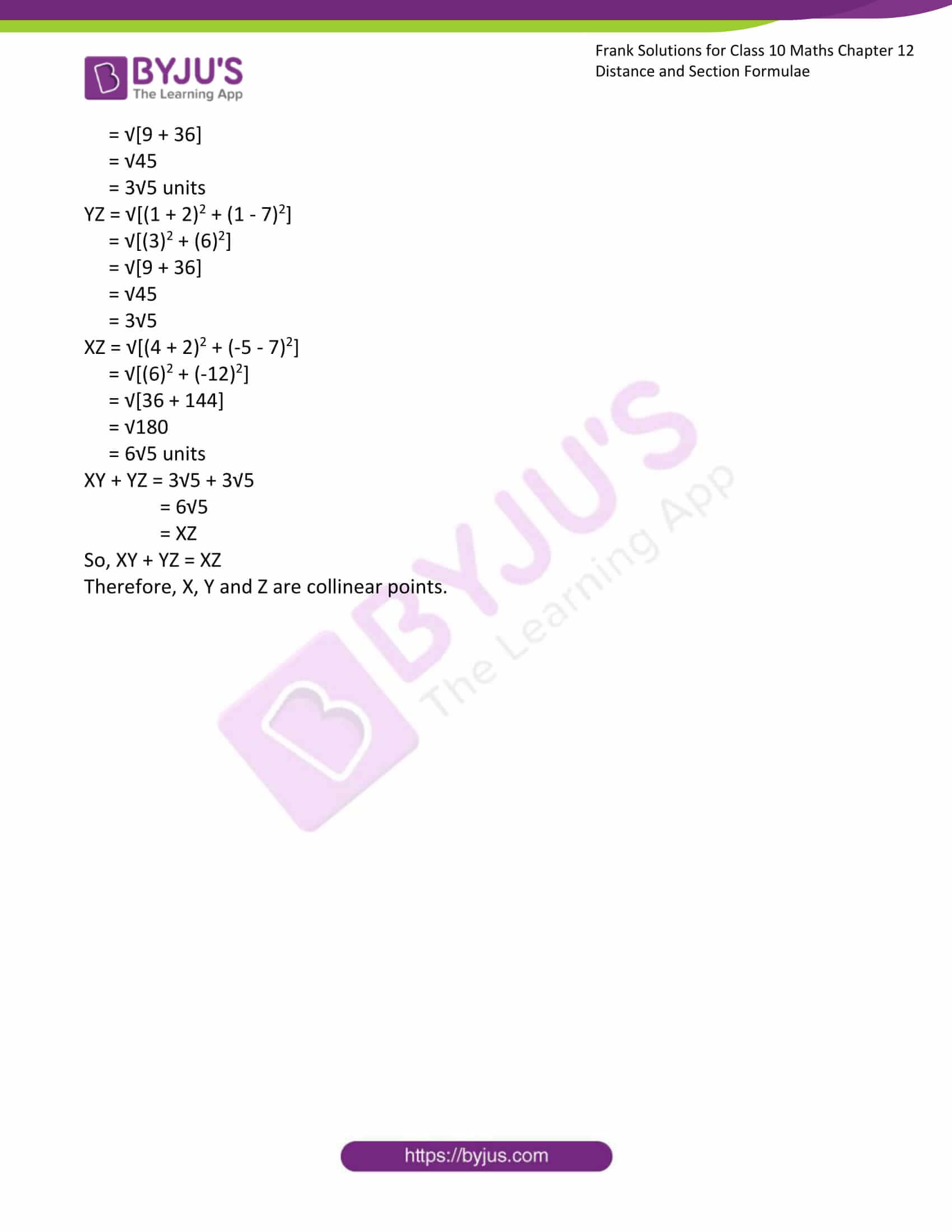### Access Answers to Frank Solutions for Class 10 Maths Chapter 12 Distance and Section Formulae

1. Find the distance between the following pairs of points in the coordinate plane:

(a) (5, -2) and (1, 5)

Solution:-

A = (5, – 2)

B = (1, 5)

AB = √((x2 – x1)2 + (y2 – y1)2

Where, x1 = x coordinate of A = 5

y1 = y coordinate of A = – 2

x2 = x coordinate of B = 1

y2 = y coordinates of B = 5

= √((1 – 5)2 + (5 + 2)2)

= √((4)2 + (7)2)

= √(16 + 49)

= √65 units

(b) (1, 3) and (3, 9)

Solution:-

A = (1, 3), B = (3, 9)

AB = √((x2 – x1)2 + (y2 – y1)2

Where, x1 = x coordinate of A = 1

y1 = y coordinate of A = 3

x2 = x coordinate of B = 3

y2 = y coordinates of B = 9

= √((3 – 1)2 + (9 – 3)2)

= √((2)2 + (6)2)

= √(4 + 36)

= √40 units

= 2√10 units

(c) (7, -7) and (2, 5)

Solution:-

A = (7, -7), B = (2, 5)

AB = √((x2 – x1)2 + (y2 – y1)2

Where, x1 = x coordinate of A = 7

y1 = y coordinate of A = -7

x2 = x coordinate of B = 2

y2 = y coordinates of B = 5

= √((2 – 7)2 + (5 + 7)2)

= √((-5)2 + (12)2)

= √(25 + 144)

= √169 units

= 13 units

(d) (4, 1) and (-4, 5)

Solution:-

A = (4, 1), B = (-4, 5)

AB = √((x2 – x1)2 + (y2 – y1)2

Where, x1 = x coordinate of A = 4

y1 = y coordinate of A = 1

x2 = x coordinate of B = -4

y2 = y coordinates of B = 5

= √((4 + 4)2 + (1 – 5)2)

= √((8)2 + (4)2)

= √(64 + 16)

= √80 units

= 4√5 units

(e) (13, 7) and (4, -5)

Solution:-

A = (13, 7), B = (4, -5)

AB = √((x2 – x1)2 + (y2 – y1)2

Where, x1 = x coordinate of A = 13

y1 = y coordinate of A = 7

x2 = x coordinate of B = 4

y2 = y coordinates of B = -5

= √((4 – 13)2 + (- 5 – 7)2)

= √((-9)2 + (-12)2)

= √(81 + 144)

= √225 units

= 15 units

2. Find the distances of the following points from the origin.

(a) (5, 12)

Solution:-

Origin A = (0, 0), B = (5, 12)

AB = √((x2 – x1)2 + (y2 – y1)2

Where, x1 = x coordinate of A = 0

y1 = y coordinate of A = 0

x2 = x coordinate of B = 5

y2 = y coordinates of B = 12

= √((5 – 0)2 + (12 – 0)2)

= √((5)2 + (12)2)

= √(25 + 144)

= √169 units

= 13 units

(b) (6, 8)

Solution:-

Origin A = (0, 0), B = (6, 8)

AB = √((x2 – x1)2 + (y2 – y1)2

Where, x1 = x coordinate of A = 0

y1 = y coordinate of A = 0

x2 = x coordinate of B = 6

y2 = y coordinates of B = 8

= √((6 – 0)2 + (8 – 0)2)

= √((6)2 + (8)2)

= √(36 + 64)

= √100 units

= 10 units

(C) (8, 15)

Solution:-

Origin A = (0, 0), B = (8, 15)

AB = √((x2 – x1)2 + (y2 – y1)2

Where, x1 = x coordinate of A = 0

y1 = y coordinate of A = 0

x2 = x coordinate of B = 8

y2 = y coordinates of B = 15

= √((8 – 0)2 + (15 – 0)2)

= √((8)2 + (15)2)

= √(64 + 225)

= √289 units

= 17 units

(d) (0, 11)

Solution:-

Origin A = (0, 0), B = (0, 11)

AB = √((x2 – x1)2 + (y2 – y1)2

Where, x1 = x coordinate of A = 0

y1 = y coordinate of A = 0

x2 = x coordinate of B = 0

y2 = y coordinates of B = 11

= √((0 – 0)2 + (11 – 0)2)

= √((0)2 + (11)2)

= √(0 + 121)

= √121 units

= 11 units

(e) (13, 0)

Solution:-

Origin A = (0, 0), B = (13, 0)

AB = √((x2 – x1)2 + (y2 – y1)2

Where, x1 = x coordinate of A = 0

y1 = y coordinate of A = 0

x2 = x coordinate of B = 13

y2 = y coordinates of B = 0

= √((13 – 0)2 + (0 – 0)2)

= √((13)2 + (0)2)

= √(169 + 0)

= √169 units

= 13 units

3. Find the distance between the following points.

(a) (p + q, p – q) and (p – q, p – q)

Solution:-

A (p + q, p – q), B (p – q, p – q)

AB = √((x2 – x1)2 + (y2 – y1)2

Where, x1 = x coordinate of A = p + q

y1 = y coordinate of A = p – q

x2 = x coordinate of B = p – q

y2 = y coordinates of B = p – q

= √[((p – q) – (p + q))2 + ((p – q) – (p – q))2]

= √[(p – q – p – q)2 + (p – q – p + q)2]

= √[(-2q)2 + (0)2]

= √4q units

(b) (sin θ, cos θ) and (cos θ, -sin θ)

Solution:-

A (sin θ, cos θ), B (cos θ, -sin θ)

AB = √((x2 – x1)2 + (y2 – y1)2

Where, x1 = x coordinate of A = sin θ

y1 = y coordinate of A = cos θ

x2 = x coordinate of B = cos θ

y2 = y coordinates of B = – sin θ

= √[(cos θ – sin θ)2 + (- sin θ – cos θ)2]

= √[cos2 θ + sin2 θ – 2 cos θ sin θ + sin2 θ + cos2 θ + 2 cos θ sin θ]

= √2 units

(c) (sec θ, tan θ) and (-tan θ, sec θ)

Solution:-

A (sec θ, tan θ), B (-tan θ, sec θ)

AB = √((x2 – x1)2 + (y2 – y1)2

Where, x1 = x coordinate of A = sec θ

y1 = y coordinate of A = tan θ

x2 = x coordinate of B = -tan θ

y2 = y coordinates of B = sec θ

= √[(- tan θ – sec θ)2 + (sec θ – tan θ)2]

= √[tan2 θ + sec2 θ – 2 tan θ sec θ + sec2 θ + tan2 θ – 2 tan θ sec θ]

= √(2 sec2 θ + 2 tan2 θ) units

(d) (sin θ – cosec θ, cos θ – cot θ) and (cos θ – cosec θ, – sin θ – cot θ)

Solution:-

A (sin θ – cosec θ, cos θ – cot θ), B (cos θ – cosec θ, – sin θ – cot θ)

AB = √((x2 – x1)2 + (y2 – y1)2

Where, x1 = x coordinate of A = sin θ – cosec θ

y1 = y coordinate of A = cos θ – cot θ

x2 = x coordinate of B = cos θ – cosec θ

y2 = y coordinates of B = – sin θ – cot θ

= √[((cos θ – cosec θ) – (sin θ – cosec θ))2 + ((- sin θ – cot θ) – (cos θ – cot θ))2]

= √[(cos θ – cosec θ – sin θ + cosec θ)2 + (- sin θ – cot θ – cos θ + cot θ)2]

= √[(cos θ – sin θ)2 + (- sin θ – cos θ)2]

= √(cos2 θ + sin2 θ – 2 cos θ sin θ + sin2 θ + cos 2 θ + 2 sin θ cos θ)

= √2 units

4. Find the distance of a point (7, 5) from another point on the x – axis whose abscissa is – 5.

Solution:-

From the question it is given that, abscissa is – 5.

Let us assume point on x – axis be (x, 0)

So, point P (-5, 0)

Point Q (7, 5)

PQ = √((7 + 5)2 + (5 – 0)2)

= √((12)2 + (5)2)

= √(144 + 25)

= √169

= 13 units

5. Find the distance of a point (13, -9) from another point on the line y = 0 whose abscissa is 1.

Solution:-

From the question it is given that point on the line y = 0 whose abscissa is 1.

So, point is P(1, 0)

Let us assume (13, -9) be point Q.

PQ = √((13 – 1)2 + (-9 – 0)2)

= √((12)2 + (-9)2)

= √(144 + 81)

= √225

= 15 units

6. Find the distance of a point (12, 5) from another point on the line x = 0 whose ordinate is 9.

Solution:-

From the question it is given that, point on the line x = 0 whose ordinate is 9.

So, point is P(0,9)

Let us assume that point (12, 5) be Q.

PQ = √((12 – 0)2 + (5 – 9)2)

= √((12)2 + (-4)2)

= √(144 + 16)

= √160

= 4√10 units

7. Find the value of a if the distance between the points (5, a) and (1, 5) is 5 units.

Solution:-

From the question it is given that,

The distance between the points (5, a) and (1, 5), assume the two points be A and B respectively.

AB = 5 units

√((5 – 1)2 + (a + 5)2) = 5

Now, squaring on both side we get,

(5 – 1)2 + (a + 5)2 = 25

(25 + 1 – 10) + (a2 + 25 + 10a) = 25

16 + a2 + 25 + 10a = 25

a2 + 10a + 16 = 25 – 25

a2 + 10a + 16 = 0

a2 + 8a + 2a + 16 = 0

Now, take out common in above terms we get,

a(a + 8) + 2(a + 8) = 0

(a + 8) (a + 2) = 0

a + 8 = 0, a + 2 = 0

a = -8, a = – 2

8. Find the value of m if the distance between the points (m, -4) and (3, 2) is 3√5 units.

Solution:-

From the question it is given that,

The distance between the points (m, -4) and (3, 2), assume the two points be A and B respectively.

AB = 3√5 units

√((m – 3)2 + (-4 – 2)2) = 3√5

Now, squaring on both side we get,

(m – 3)2 + (-4 – 2)2 = 45

(m2 + 9 – 6m) + (16 + 4 + 16) = 45

m2 + 9 – 6m + 36 = 45

By transposing we get,

m2 – 6m + 45 – 45 = 0

m2 – 6m = 0

Now, take out common in above terms we get,

m(m – 6) = 0

m = 0, m – 6 = 0

m = 0, m = 6.

9. Find the relation between a and b if the point P(a, b) is equidistant from A(6, -1) and B(5, 8).

Solution:-

From the question it is given that, point A (6, -1), point B (5, 8)

Then, P(a, b) is equidistant

So, PA = PB

PA2 = PB2

(a – 6)2 + (b + 1)2 = (a – 5)2 + (b – 8)2

We know that, (a + b)2 = a2 + 2ab + b2 and (a – b)2 = a2 – 2ab + b2

a2 + 36 – 12a + b2 + 1 + 2b = a2 + 25 – 10a + b2 + 64 – 16b

By transposing we get,

a2 – a2 + 37 – 89 – 12a + 10a + b2 – b2 + 2b + 16b = 0

– 52 – 2a + 18b = 0

Divide both side by 2 we get,

-2a/2 + 18b/2 – 52/2 = 0

-a + 9b – 26 = 0

a = 9b – 26

10. Find the relation between x and y if the point M(x, y) is equidistant from R(0, 9) and T(14, 11).

From the question it is given that, point R(0, 9) and T(14, 11)

Then, M(x, y) is equidistant from R and T.

So, MR = MT

MR2 = MT2

(x – 0)2 + (y – 9)2 = (x – 14)2 + (y – 11)2

We know that, (a + b)2 = a2 + 2ab + b2 and (a – b)2 = a2 – 2ab + b2

x2 + y2 + 81 – 18y = x2 + 196 – 28x + y2 + 121 – 22y

By transposing we get,

x2 – x2 + y2 – y2 + 81 – 317 – 18y + 22y + 28x = 0

– 236 + 4y + 28x = 0

Divide both side by 4 we get,

4y/4 + 28x/4 – 236/4 = 0

y + 7x – 59 = 0

11. Find the distance between P and Q if P lies on the y – axis and has an ordinate 5 while Q lies on the x – axis and has an abscissa 12.

Solution:-

From the question it is given that,

P lies on the y – axis and has an ordinate 5.

Then, point P (0, 5)

And also in the question it is given that, Q lies on the x – axis and has an abscissa 12.

So, point Q (12, 0)

We know that, PQ = √((x2 – x1)2 + (y2 – y1)2

Where, x1 = x coordinate of P = 0

y1 = y coordinate of Q = 5

x2 = x coordinate of P = 12

y2 = y coordinates of Q = 0

= √((12 – 0)2 + (0 – 5)2)

= √((144) + (25))

= √(169)

= 13 units

12. P and Q are two points lying on the x – axis and the y – axis respectively. Find the coordinates of P and Q if the difference between the abscissa of P and the ordinates of Q is 1 and PQ is 5 units.

Solution:-

From the question it is given that,

P and Q are two points lying on the x – axis and the y – axis respectively.

The difference between the abscissa of P and the ordinates of Q is 1 and PQ is 5 units.

Let us assume abscissa of P be ‘a’ the ordinate of Q is a – 1.

So, P(a, 0), Q(0, a – 1)

Then, √[(x – 0)2 + (0 – x + 1)2] = 5

Now, squaring on both side we get,

x2 + x2 + 1 – 2x = 25

2x2 + 1 – 2x = 25

By transposing we get,

2x2 + 1 – 2x – 25 = 0

2x2 – 2x – 24 = 0

Divide both side by 4 we get,

2x2/2 – 2x/2 – 24/2 = 0

x2 – x – 12 = 0

x2 – 4x + 3x – 12 = 0

Now, take out common in above terms we get,

x(x – 4) + 3(x – 4) = 0

(x – 4) (x + 3) = 0

x – 4 = 0, x + 3 = 0

x = 4, x = -3

Therefore, the coordinates of P are (4, 0) or (-3, 09)

And coordinates of Q are (0, 3) or (0, -4).

13. Find the point on the x – axis equidistant from the points (5, 4) and (-2, 3).

Solution:-

Let us assume the point on x – axis be Q (x, 0) and point A(5, 4), point B (-2, 3)

From the question it is given that, QA = QB

So, QA2 = QB2

(x – 5)2 + (0 – 4)2 = (x + 2)2 + (0 – 3)2

x2 + 25 – 10x + 16 = x2 + 4 + 4x + 9

On simplification we get,

– 14x + 28 = 0

14x = 28

x = 28/14

x = 2

Therefore, point on x – axis is (2, 0)

14. A line segment of length 10 units has one end at A (-4, 3). If the ordinate of the other end B is 9, find the abscissa of this end.

Solution:-

From the question it is given that,

Length of line segment i.e. AB = 10 units

Point A is (-4, 3)

Let us assume point B is (y, 9)

Then,

√[(-4 – x)2 + (3 – 9)2] = 10

Now, squaring on both side we get,

(-4 – x)2 + (3 – 9)2 = 100

16 + x2 + 8x + 36 = 100

By transposing we get,

16 + 36 – 100 + x2 + 8x = 0

x2 + 8x – 48

x2 + 12x – 4x – 48 = 0

Now, take out common in above terms we get,

x(x + 12) – 4(x + 12) = 0

(x – 4) (x + 12) = 0

x – 4 = 0, x + 12 = 0

x = 4, x = 12

Hence, the abscissa of other end is 4 or – 12.

15. Prove that the following sets of points are collinear:

(a) (5, 5), (3, 4), (-7, -1)

Solution:-

Three or more points that lie on the same line are called collinear points.

Let us assume X (5, 5), Y (3, 4) and Z (-7, -1)

Then,

XY = √[(5 – 3)2 + (5 – 4)2]

= √[(2)2 + (1)2]

= √[4 + 1]

= √5 units

YZ = √[(3 + 7)2 + (4 + 1)2]

= √[(10)2 + (5)2]

= √[100 + 25]

= √100

= 5√5 units

XZ = √[(5 + 7)2 + (5 + 1)2]

= √[(12)2 + (6)2]

= √[144 + 36]

= √180

= 6√5 units

XY + YZ = √5 + 5√5

= 6√5

= XZ

So, XY + YZ = XZ

Therefore, X, Y and Z are collinear points.

(b) (5, 1), (3, 2), (1, 3)

Solution:-

Three or more points that lie on the same line are called collinear points.

Let us assume X (5, 1), Y (3, 2) and Z (1, 3)

Then,

XY = √[(5 – 3)2 + (1 – 2)2]

= √[(2)2 + (-1)2]

= √[4 + 1]

= √5 units

YZ = √[(3 – 1)2 + (2 – 3)2]

= √[(2)2 + (-1)2]

= √[4 + 1]

= √5 units

XZ = √[(5 – 1)2 + (1 – 3)2]

= √[(4)2 + (-2)2]

= √[16 + 4]

= √20

= 2√5 units

XY + YZ = √5 + √5

= 2√5

= XZ

So, XY + YZ = XZ

Therefore, X, Y and Z are collinear points.

(c) (4, -5), (1, 1), (-2, 7)

Solution:-

Three or more points that lie on the same line are called collinear points.

Let us assume X (4, -5), Y (1, 1) and Z (-2, 7)

Then,

XY = √[(4 – 1)2 + (-5 – 1)2]

= √[(3)2 + (-6)2]

= √[9 + 36]

= √45

= 3√5 units

YZ = √[(1 + 2)2 + (1 – 7)2]

= √[(3)2 + (6)2]

= √[9 + 36]

= √45

= 3√5

XZ = √[(4 + 2)2 + (-5 – 7)2]

= √[(6)2 + (-12)2]

= √[36 + 144]

= √180

= 6√5 units

XY + YZ = 3√5 + 3√5

= 6√5

= XZ

So, XY + YZ = XZ

Therefore, X, Y and Z are collinear points.